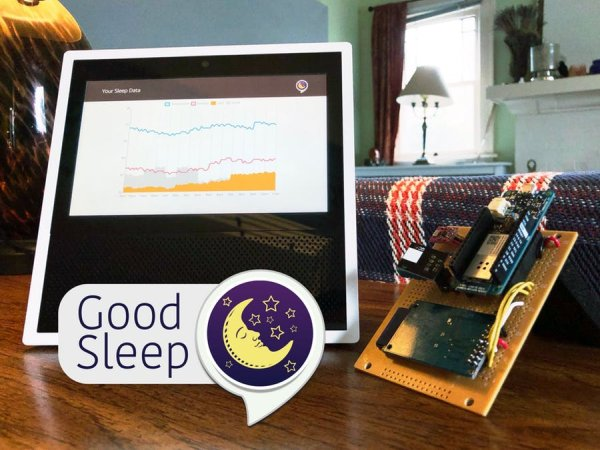# Troubleshooting a 32kHz Crystal Oscillator

For Project Whack a Mole I need a 32.768kHz crystal oscillator. I found this circuits on the Interwebs and gave it a try:It wouldn’t go. I messed about changing component values for while, then decided to actually try to understand the circuit. Now for an oscillator to work, we need an amplifier with a gain of greater than 1, and a phase shift of 360 degrees to get positive feedback.

The circuit above is an amplifier, with the crystal network connected between the collector output and base input. We get half of the 360 degree phase shift by using a common emitter topology, which is an inverting amplifier. So the crystal network must provide the other 180 degrees. On a good day. If it’s working.

First problem – the transistor was saturated, with Vc stuck near 0V. For an oscillator to start noise gets amplified, filtered by the crystal, amplified again etc. I reasoned that if the amplifier wasn’t biased to be linear, the oscillations couldn’t build up. So I reduced the collector resistor to 6.8k, and changed the the base bias resistor to 1.8M to get the collector voltage into a linear region. So now we have Vc=3.2V with a 5V supply.

But it still wouldn’t go. On a whim I adjusted the supply voltage up and then down and found it would start with a supply voltage beneath 3V, but not any higher. Huh?

Much fiddling with pencil and paper followed. Time for a LT Spice simulation of the “AC model” of the circuit:

I’ve “opened the loop”, to model the collector driving the crystal network which then drives the base impedance. On the left is a voltage source and 6.8k resistor that represents the collector driving the 330k resistor and an equivalent model of the crystal.

READ  American and 2 Japanese Physicists Share Nobel for Work on LED Lights

The values Lm, Cm, Rm, are the “motional” parameters. They are what the mechanical properties of the crystal look like to this circuit. The values are amazing, unrealizable if you are used to regular electronic parts. I found Cm = 1fF (1E-15 Farads, or 0.001 pF) in a 32kHz crystal data sheet, then solved f=1/(2*pi*sqrt(LC)) for Lm to get the remarkable value of 24,000 Henrys. Wow.

Phase Shift

With Vcc=5V, we have Vc=3.2V, so a collector current Ic = (5-3.2)/6800 = 0.265mA. I’m using a small signal transistor model, with the emitter resistance re=26/Ic = 26/0.265 = 100 ohms. The effective impedance looking into the base rb=beta*re = 100*100 = 10k ohms (2N3904’s have a minimum beta of 100).